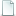## Stanford EE364A - Convex Optimization I - Boyd Stephen BoydStanford-EE364A-ConvexOptimizationI-Boyd (41 files)lectures/Lecture 1 _ Convex Optimization I (Stanford)-McLq1hEq3UY.mp4 220.43MBlectures/Lecture 2 _ Convex Optimization I (Stanford)-P3W_wFZ2kUo.mp4 221.55MBlectures/Lecture 3 _ Convex Optimization I (Stanford)-kcOodzDGV4c.mp4 212.16MBlectures/Lecture 4 _ Convex Optimization I (Stanford)-lEN2xvTTr0E.mp4 201.78MBlectures/Lecture 5 _ Convex Optimization I (Stanford)-Ry5i8DGZrJs.mp4 267.03MBlectures/Lecture 6 _ Convex Optimization I (Stanford)--T9cloGG_80.mp4 189.37MBlectures/Lecture 7 _ Convex Optimization I-VxQ8VHm1Ci4.mp4 250.32MBlectures/Lecture 8 _ Convex Optimization I (Stanford)-FJVmflArCXc.mp4 208.74MBlectures/Lecture 9 _ Convex Optimization I (Stanford)-3Q9mMluX3Gw.mp4 208.89MBlectures/Lecture 10 _ Convex Optimization I (Stanford)-gH13lxieYFU.mp4 212.46MBlectures/Lecture 11 _ Convex Optimization I (Stanford)-GxK04B9SVg4.mp4 258.62MBlectures/Lecture 12 _ Convex Optimization I (Stanford)-mNzu42FrlHo.mp4 354.99MBlectures/Lecture 13 _ Convex Optimization I (Stanford)-FkPLteYMK40.mp4 216.83MBlectures/Lecture 14 _ Convex Optimization I (Stanford)-ZmvQ7GQ_gPg.mp4 191.39MBlectures/Lecture 15 _ Convex Optimization I (Stanford)-sTCtkkqrY8A.mp4 209.23MBlectures/Lecture 16 _ Convex Optimization I (Stanford)-Ap8LGbCVx4I.mp4 243.10MBlectures/Lecture 17 _ Convex Optimization I (Stanford)-StlHUwd_AgM.mp4 259.41MBlectures/Lecture 18 _ Convex Optimization I (Stanford)-oMRVDILkpUI.mp4 318.69MBlectures/Lecture 19 _ Convex Optimization I (Stanford)-HZW-9Ar0iVc.mp4 209.00MBslides/approx.pdf 349.43kBslides/barrier.pdf 190.29kBslides/chance_constr.pdf 90.33kBslides/conclusions.pdf 28.40kBslides/convexjl_tutorial.pdf 80.30kBslides/cvx_lecture_slides.pdf 96.88kBslides/cvx_tutorial.pdf 131.02kBslides/duality.pdf 122.05kBslides/equality.pdf 100.63kBslides/examples.pdf 138.51kBslides/filters.pdf 158.14kBslides/functions.pdf 167.78kBslides/geom.pdf 233.19kBslides/intro.pdf 63.78kBslides/l1_ext_slides.pdf 907.49kBslides/l1_slides.pdf 185.07kBslides/num-lin-alg.pdf 75.41kBslides/problems.pdf 218.36kBslides/sets.pdf 148.28kBslides/stat.pdf 111.33kBslides/stoch_prog.pdf 87.29kBslides/unconstrained.pdf 362.81kB
Type: Course
Tags: Optimization, Math

Bibtex:
```@article{,
title= {Stanford EE364A - Convex Optimization I - Boyd},
journal= {},
author= {Stephen Boyd},
year= {2008},
url= {https://web.stanford.edu/class/ee364a/},
abstract= {Catalog description
Concentrates on recognizing and solving convex optimization problems that arise in applications. Convex sets, functions, and optimization problems. Basics of convex analysis. Least-squares, linear and quadratic programs, semidefinite programming, minimax, extremal volume, and other problems. Optimality conditions, duality theory, theorems of alternative, and applications. Interior-point methods. Applications to signal processing, statistics and machine learning, control and mechanical engineering, digital and analog circuit design, and finance.

Course objectives
to give students the tools and training to recognize convex optimization problems that arise in applications to present the basic theory of such problems, concentrating on results that are useful in computation to give students a thorough understanding of how such problems are solved, and some experience in solving them to give students the background required to use the methods in their own research work or applications

Videos
1. Introduction
2. Convex sets
3. Convex functions
4. Convex optimization problems
5. Duality
6. Approximation and fitting
7. Statistical estimation
8. Geometric problems
9. Numerical linear algebra background
10. Unconstrained minimization
11. Equality constrained minimization
12. Interior-point methods
13. Conclusions
},
keywords= {Optimization, Math},
terms= {}
}

```

Send Feedback